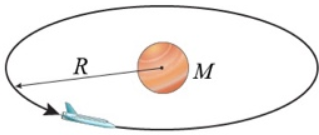# Problem: An intergalactic spaceship arrives at a distant planet that rotates on its axis with a period of T = 45 hours. The spaceship enters a geosynchronous orbit at a distance of R = 8.7 × 108 m Part (a) From the given information, write an equation for the mass of the planet. Part (b) Calculate the mass of the planet in kilograms.

###### FREE Expert Solution

Centripetal forces are equal to gravitational forces.

$\overline{){\mathbf{\Sigma }}{{\mathbf{F}}}_{{\mathbf{C}}}{\mathbf{=}}{\mathbf{m}}{{\mathbf{a}}}_{{\mathbf{C}}}}$

(a)

Centripetal force:

$\overline{){{\mathbf{F}}}_{{\mathbf{C}}}{\mathbf{=}}\frac{\mathbf{G}\mathbf{M}\mathbf{m}}{{\mathbf{R}}^{\mathbf{2}}}}$

Centripetal acceleration:

$\overline{){{\mathbf{a}}}_{{\mathbf{C}}}{\mathbf{=}}\frac{{\mathbf{v}}^{\mathbf{2}}}{\mathbf{R}}}$

Substituting:

$\begin{array}{rcl}\frac{\mathbf{GM}\overline{)\mathbf{m}}}{{\mathbf{R}}^{\mathbf{2}}}& \mathbf{=}& \frac{\overline{)\mathbf{m}}{\mathbf{v}}^{\mathbf{2}}}{\mathbf{R}}\\ \mathbf{v}& \mathbf{=}& \sqrt{\frac{\mathbf{G}\mathbf{M}}{\mathbf{R}}}\end{array}$

The velocity, v is:

$\overline{){\mathbf{v}}{\mathbf{=}}\frac{\mathbf{2}\mathbf{\pi }\mathbf{R}}{\mathbf{T}}}$

82% (264 ratings)###### Problem Details

An intergalactic spaceship arrives at a distant planet that rotates on its axis with a period of T = 45 hours. The spaceship enters a geosynchronous orbit at a distance of R = 8.7 × 108Part (a) From the given information, write an equation for the mass of the planet.

Part (b) Calculate the mass of the planet in kilograms.